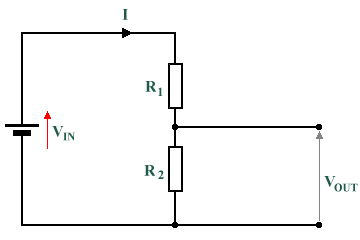# Answers to Problems - The Potential Divider

1. The diagram below shows a circuit divider consisting of two resistors in series with a supply voltage. Statements (a) through (e) give values for each element of the circuit except one. For each statement, calculate the missing value.

a) VIN = 60 V,     R1 = 6 Ω,     R2 = 4 Ω

b) VIN = 20 V,     R1 = 5 Ω,     R2 = 5 Ω

c) R1 = 10 Ω,     R2 = 5 Ω,     VOUT = 10 V

d) VIN = 50 V,     R1 = 10 Ω,     VOUT = 10 V

e) VIN = 20 V,     R2 = 4 Ω,     VOUT = 5 VA potential divider consisting of two resistors in series with a supply voltage

a) Find VOUT:

 VOUT  = VIN  ×  R2 = 60  ×  4 =  24 V R2  +  R1 4  +  6

b) Find VOUT:

 VOUT  = VIN  ×  R2 = 20  ×  5 =  10 V R2  +  R1 5  +  5

c) Find VIN:

 VIN  =  VOUT  × R2  +  R1 =  10  × 5  +  10 =  30 V R2 5

d) Find R2:

 R2 = VOUT R2  +  R1 VIN

R2  ×  VIN  =  (R2  +  R1)  ×  VOUT

R2  ×  50  =  R2  ×  10  +  100

R2  ×  40  =  100

R2  =  100  ÷  40  =  2.5 Ω

e) Find R1:

 R2 = VOUT R2  +  R1 VIN

R2  ×  VIN  =  (R2  +  R1)  ×  VOUT

80  =  20  +  R1  ×  5

R1  ×  5  =  60

R1  =  60  ÷  5  =  12 Ω